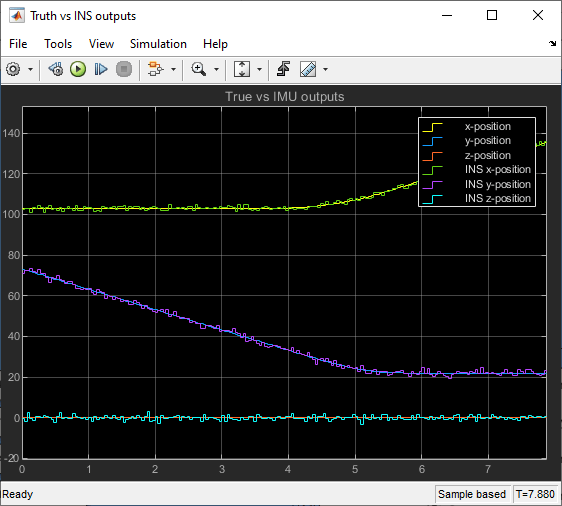# Simulate INS Block

In this example, you simulate an INS block by using the pose information of a vehicle undertaking a left-turn trajectory.

First, you load the trajectory information of the vehicle to the workspace.

`load leftTurnTrajectory.mat`

You notice that seven new variables appear in MATLAB workspace.

• `dt` — The time step size of 0.4 seconds.

• `t` — The total time span of 7.88 seconds.

• `vehPos`, `vehVel`, `vehAcc`, `vehOrient`, `vehAngVel` — The history of position, velocity, acceleration, orientation, and angular velocity, each specified as a 198-by-3 matrix, where 198 is the total number of steps.

Next, you open the Simulink model.

`open simulateINS.slx`The model contains three parts: the data importing part, the INS block, and the scope block to compare the true positions with the INS outputs.

The data importing part imports the vehicle trajectory data into Simulink using the From Workspace block. You use a helper function `helperFromWorkspace`, attached in the example folder, to convert the trajectory data into a structure format required by the From Workspace block.

### Run the Model

`resulsts = sim('simulateINS');`